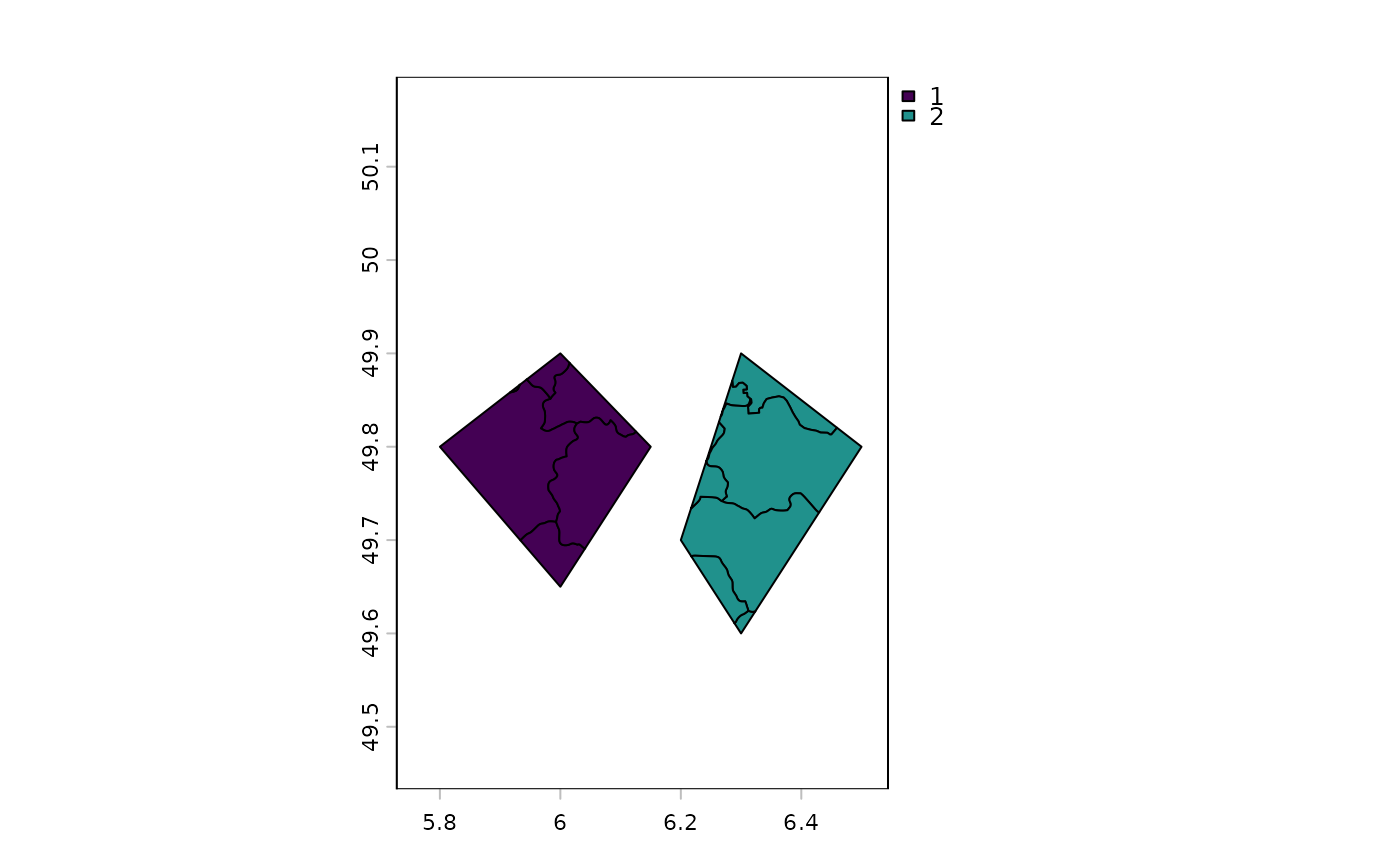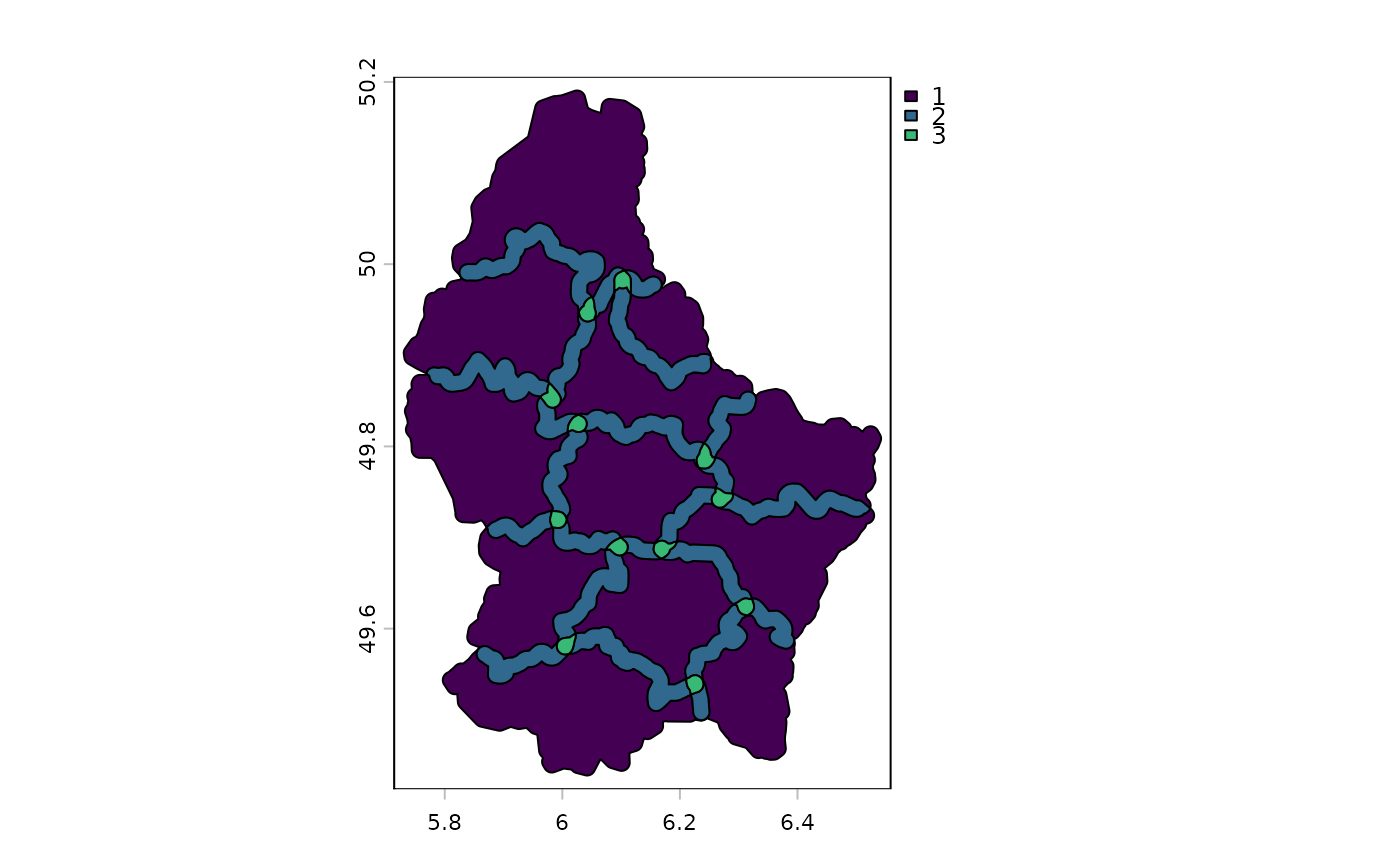Overlapping polygons (between, not within, objects) are intersected. Union for lines and points simply combines the two data sets; without any geometric intersections. This is equivalent to c. Attributes are joined. See c if you want to combine polygons without intersection.

If x and y have a different geometry type, a SpatVectorCollection is returned.

If a single SpatVector is supplied, overlapping polygons are intersected. Original attributes are lost. New attributes allow for determining how many, and which, polygons overlapped.

SpatExtent: Objects are combined into their union; this is equivalent to +.

# S4 method for SpatVector,SpatVector
union(x, y)

# S4 method for SpatVector,missing
union(x, y)

# S4 method for SpatExtent,SpatExtent
union(x, y)

## Arguments

x

SpatVector or SpatExtent

y

Same as x or missing

## Value

SpatVector or SpatExtent

intersect

merge and mosaic to union SpatRaster objects.

crop and extend for the union of SpatRaster and SpatExtent.

merge for merging a data.frame with attributes of a SpatVector.

aggregate to dissolve SpatVector objects.

## Examples

e1 <- ext(-10, 10, -20, 20)
e2 <- ext(0, 20, -40, 5)
union(e1, e2)
#> SpatExtent : -10, 20, -40, 20 (xmin, xmax, ymin, ymax)

#SpatVector
v <- vect(system.file("ex/lux.shp", package="terra"))
v <- v[,3:4]
p <- vect(c("POLYGON ((5.8 49.8, 6 49.9, 6.15 49.8, 6 49.65, 5.8 49.8))",
"POLYGON ((6.3 49.9, 6.2 49.7, 6.3 49.6, 6.5 49.8, 6.3 49.9))"), crs=crs(v))
values(p) <- data.frame(pid=1:2, value=expanse(p))
u <- union(v, p)
plot(u, "pid")b <- buffer(v, 1000)

u <- union(b)
u\$sum <- rowSums(as.data.frame(u))
plot(u, "sum")